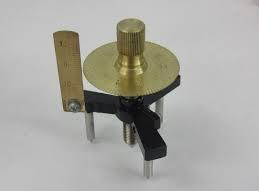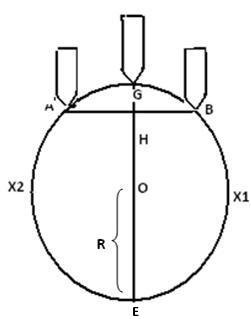you are here->home->Physics->Class 11->Spherometer
Spherometer

# Objective

Our objectives are;

• To determine the thickness of a glass plate using a spherometer.
•  To determine the radius of curvature of a given spherical surface using a spherometer.

# Theory

A spherometer works on the principle of the micrometer screw. It is used to measure either very small thickness of flat materials like glass or the radius of curvature of a spherical surface thus getting its name.## Parts of a Spherometer

A spherometer consists of a metallic tripod framework supported on three fixed legs of equal lengths. A screw passes through the centre of the tripod frame, parallel to the three legs. A largeP)circular disc graduated with 100 equal parts is attached to the top of the screw. A small vertical scale known as the Pitch scale (P) with the scale reading divided into millimeters is fixed at one end of the tripod frame.

## Thickness of a Glass Strip

The thickness of a glass strip  can be represented by;Where, 'n' is the number of complete rotation made by the circular disc.

'P' is the pitch, which is the distance moved by the middle screw per revolution.

'x' is the number of additional circular scale divisions in excess of complete rotations.

'L.C' is the least count of spherometer.

## Least Count of the Spherometer

The pitch of the screw needs to be measured first.  Find the value of 1 division on the pitch scale. Then bring the zero of the circular scale opposite to the pitch scale and rotate the screw by 'n' complete rotations. Note the distance 'd' moved by the screw on the pitch scale. The pitch of the screw is given by;If there are N divisions in the circular scale on the circumference of the disc then;## Measuring the Radius of Curvature of the Concave Surface

Let the figure represent the vertical section of the sphere of which the given surface forms a part, the vertical section passes through one of the outer legs 'A', the central screw 'G' and the centre of the sphere 'O'.The third leg 'C' is not visible.

Let, the height of central screw above the plane ABC, which is measured by the spherometer (h is called Sagitta.)LetButwhere 'l' is the distance between  any of the two legs.

Therefore,Since,## Learning Outcomes

• Students know the working of a spherometer.
• Students know the least count of spherometer.
• They know the equations for thickness of glass plate and radius of curvature of the concave surface.

Cite this Simulator: